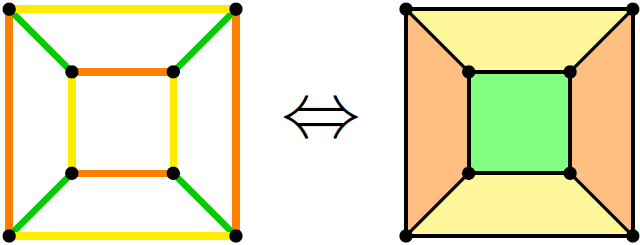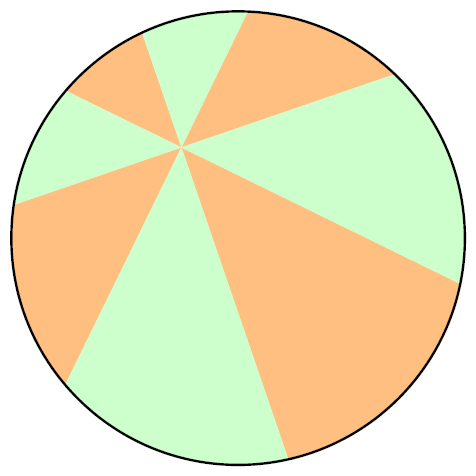## 水贴：茱莉亚集合动图两幅

π节/白色情人节快乐！我是渡鸦，最近没什么时间写新的文，以后可能要不定期地鸽下去了咕咕。今天意外地翻到了自己一年前做过的动图，所以滥竽充数地发一篇看图贴了，希望大家理解。

## 功败垂成的四色定理：被埋没的史话## 求导证明披萨定理## π的恒等式一则，和灯塔

$\frac1{1^3}-\frac1{3^3}+\frac1{5^3}-\frac1{7^3}+\frac1{9^3}\pm\cdots=\frac{\pi^3}{32}$